##### Actions

(diff) ← Older revision | Latest revision (diff) | Newer revision → (diff)in which the nodes (cf. Node)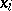and the weights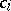are so selected that the formula is exact for the functions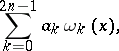whereare given linearly independent functions (the integration limits may well be infinite). The formula was introduced by C.F. Gauss  for,,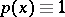. He obtained the following formula, which is exact for an arbitrary polynomial of degree not exceeding: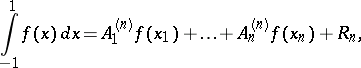where the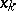are the roots of the Legendre polynomial (cf. Legendre polynomials)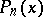, whileand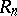are defined by the formulas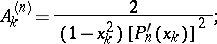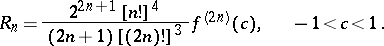The formula is used whenever the integrand is sufficiently smooth, and the gain in the number of nodes is substantial; for instance, ifis determined from expensive experiments or during the computation of multiple integrals as repeated integrals. In such practical applications a suitable choice of the weight function and of the functionsis very important.

Tables of nodes in Gauss' quadrature formula are available for wide classes of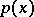and; in particular for,up to.

If,, Gauss' quadrature formula is employed in standard integration programs with an automatic step selection as a method of computing integrals by subdivision of subsegments .

How to Cite This Entry: